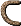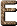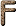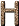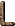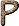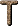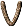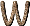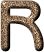R-Branch A set of lines in the spectra of molecules corresponding to unit decreases in rotational energy. [H76] R Galaxy In the Yerkes9 1974 system, a system showing rotational symmetry, without clearly marked spiral or elliptical structure (formerly called D galaxy). [H76] r-Process The capture of neutrons on a very rapid time scale (i.e., one in which a nucleus can absorb neutrons in rapid succession, so that regions of great nuclear instability are bridged), a theory advanced to account for the existence of all elements heavier than bismuth (up to A298) as well as the neutron-rich isotopes heavier than iron. The essential feature of the r-process is the release of great numbers of neutrons in a very short time (less than 100 seconds). The presumed source for such a large flux of neutrons is a supernova, at the boundary between the collapsing neutron star and the ejected material. However, other proposed sources have included such things as supernova shocks and black-hole-neutron-star collisions. The heavier r-process elements are synthesized at a temperature of about 109 K and an assumed neutron density of 1020-1030 per cm3. The r-process is terminated by neutron-induced fission. The existence of 244Pu (half-life 82 million years) in the early solar system shows that at least one r-process event had occurred in the Galaxy just before the formation of the solar system. [H76] R Star Stars of spectral type R are stars with spectral characteristics similar to those of K stars except that molecular bands of C2, CN, and CH are present instead of TiO bands. [H76] R Zones Regions in the solar corona in which short-lived radiofrequency variations are observed. [H76] Rabi frequency this is the frequency at which atomic population is coherently transferred from one state to another by a resonant radiation field; it is named after its discoverer I. Rabi. It plays a central role in atom-field interactions, showing up in emitted light spectra and in time dependence. [D89] Rad Unit of radiation, equal to 100 ergs of ionizing energy absorbed per gram of absorber. [H76] RADAR Radio Detection and Ranging. [LLM96] Radial Pulsation Periodic expansion and contraction of a star that may be merely an optical effect of recession. [A84] Radial Velocity (a) Velocity along the line of sight toward (-) or away from (+) the observer. [H76] (b) The speed at which an object moves toward or away from us. It can be measured from a star's spectrum: a star moving toward us has a blueshifted spectrum, and a star moving away from us has a redshifted spectrum. The larger the blueshift or redshift, the larger the radial velocity. The present radial-velocity champion is a star in the constellation Lacerta named Giclas 233-27, which moves toward us at 583 kilometers per second. [C95] Radian (a) A measure of angular distance; 2radians equals 360 degrees. [Silk90] (b) A unit of angular measure equal to the angle subtended at the centre of a circle by an arc the length of which is equal to the radius. The name is reputed to have been made up by James Thomson in an examination paper in Belfast about 1870 and it was first published in 1873. There are 2radians in a circle, i.e. one radian is equal to 57.29578°. The angle/4 is called an octant and a thousandth of a radian is sometimes called a mil, which is equal to 3 minutes 26.5 seconds of arc. [JM92] Radiant (a) The point in the sky from which a meteor shower appears to emanate. [A84] (b) The convergent point toward which the stars in a moving cluster appear to travel, or from which the meteors in a shower seem to radiate. (also called the vertex) [H76] Radiation The energy carried by waves or particles. [G99] Radiation Damping A decrease in the amplitude of an oscillation due to the emission of energy by radiation. [H76] Radiation Era The era from about 10 sec to about 1012 sec after the Big Bang, when the temperature had dropped to 109K and the rate of electron-positron pair annihilation exceeded the rate of their production, leaving radiation the dominant constituent of the universe. At t = 200 sec, nucleosynthesis began rather abruptly and virtually all Deuterium was synthesized to Helium. The radiation era was followed by the matter era. [H76] Radiation Length The mean distance traveled by a relativistic particle in a given medium before its energy is reduced by a factor e by its interaction with matter. [H76] Radiation Pressure The transfer of momentum by electromagnetic radiation incident on a surface: prad = (4/3)T4 / c. [H76] Radiation Temperature The temperature that a blackbody of similar dimensions would have that radiated the same intensity at the same frequency. [H76] Radiative Braking The slowing down of rotation of a star due to radiation. [H76] Radiative Capture Capture of a free electron by an ion with the subsequent emission of an X-ray (or gamma-ray) photon (also called Radiative Recombination ). [H76] Radiative Equilibrium In a star, represents an even process by which energy (heat) is transferred from the core to the outer surface without affecting the overall stability of the star. [A84] Radiative Recombination See radiative capture. [H76] Radiative Transfer The process by which radiation travels through a medium. [LB90] Radiative Viscosity The friction produced by the collisions between matter and radiation. [LB90] Radio Electromagnetic radiation with the lowest energy and longest wavelength. Unlike visible light, radio waves penetrate dust and can be detected from throughout the Galaxy. [C95] radio astronomy the astronomy associated with radio observations of celestial objects. The waveband extends from low radio frequencies (10 MHz,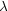: 30 m) to centimeter and millimeter wavelengths. At the low frequency end of the range, the limit is imposed by the Earth's ionosphere and at the upper end by water vapor absorption in the atmosphere. Within this waveband, many sophisticated radio telescope systems have been constructed, either using single dishes or combining them in arrays using the principles of aperture synthesis and interferometry to obtain high angular resolution. [D89] Radio Astronomy Study of the universe at the radio wavelengths of electromagnetic energy. [F88] Radio Galaxy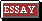A galaxy that is extremely luminous at radio wavelengths. A radio galaxy is usually a giant elliptical - the largest galaxy in a cluster - and is a strong emitter of synchrotron radiation. M87 and M82 are examples. [H76] Radio Interferometer Type of radio telescope that relies on the use of two or more aerials at a distance from each other to provide a combination of signals from one source which can be analyzed by computer. Such an analysis results in a resolution that is considerably better than that of a parabolic dish aerial by itself because of the greater effective diameter. [A84] Radio Lobes Extended regions of diffuse radio emission, often dumbbell shaped, that surround a radio galaxy. [Silk90] radio map of the sky Celestial chart depicting sources and intensities of radio emission. [A84] Radio Recombination Lines See spectral lines. Radio recombination lines are the result of electron transitions between high-n (n > 50) levels in an atom or ion. [H76] Radio Scintillation The scintillation in received radio emission; the equivalent of "twinkling" in visible light from the stars. [A84] Radio Source A source of extraterrestrial radio radiation. The strongest known is Cas A, followed by Cyg A and the Crab Nebula (Tau A) (the capital letters following the name of a constellation refer to the radio sources of the constellation, A being the strongest source). Radio sources are divided into two main categories: Class I, those associated with our Galaxy (which is a weak radio source), and Class II, extragalactic sources. Most radio sources are galaxies, supernova remnants, or H II regions. [H76] Radio Source Counts The integral number of radio sources per unit solid angle whose measured flux density at the operating frequency of a radio telescope exceeds a certain given value; plot of log N (number of sources) versus log S (where S is in flux units). [H76] Radio Stars Stars with detectable emission at radio wavelengths. They include pulsars, flare stars, some infrared stars, and some X-ray stars. [H76] Radio Telescope Non-optical telescope (of various types) which, instead of focusing light received from a distant object, focuses radio signals onto a receiver-amplifier. [A84] Radio Window The wavelength range between a few millimeters and about 20 meters within which Earth's atmosphere is transparent to radiation. [H76] Radioactivity (a) Emission of particles by unstable elements as they decay. [F88] (b) The spontaneous disintegration of unstable atomic nuclei. All natural radioactive elements heavier than lead are daughter products of either 232Th (half-life 1.39 × 1010 yr), 235U (half-life 7.13 × 108 yr), or 238U (half-life 4.51 × 109 yr). The radioactive output of the Earth averages 1.7 ergs g-1 yr-1. [H76] Radiocarbon Dating Determination of the age of a substance containing radioactive carbon by means of its radioactive half-life. [F88] Radiometer A device that detects radio waves from space and measures their direction. [LB90] Radiometric Dating Determination of the age of objects - e.g., earth and moon rocks - by means of the half-life of the unstable elements they contain. [F88] Radiosonde A sounding balloon used to transmit information on Earth's upper atmosphere. [H76] Radiotelescopes Sensitive radio antennae employed to detect the radio energy emitted by nebulae, galaxies, pulsars, etc. [F88] Radius In astronomy, an old instrument for measuring the angular distance between two celestial objects. [A84] Radius Vector In astronomy, an imaginary line connecting the center of an orbiting body with the centre of the body (or point) that it is orbiting. [A84] RAM Random access memory. A silicon micro-chip capable of temporary storage of information in the form of binary digits, either 0 or 1, and enabling rapid access to any part of its storage area. [McL97] Ram Pressure Motion of a blunt body at supersonic velocity through an ambient gaseous medium causes a strong drag or ram pressure to be exerted on the body. In the case of a galaxy moving through the intergalactic gas, the ram pressure is capable of stripping the galaxy of much of its interstellar gas. [Silk90] Raman Effect (a) In spectroscopy, the change in the wavelength of light scattered by molecules. [A84] (b) The change of wavelength on scattering. It arises from radiation exciting (or de-exciting) atoms or molecules from their initial states. [H76] Ramsauer Effect An anomalously large mean free path for low-energy electrons. [H76] Random Walk If a point experiences successive displacements such that each displacement is in a random direction and of a length also governed by a frequency distribution, then the point is said to experience a random walk. It is a law of statistical behavior closely allied to Brownian motion and the diffusion of molecules. It can be proved that the root mean square displacement experienced in N mean free paths is related to the diffusion coefficient by Drms =N. [H76] Ranger Spaceprobes Series of 9 US spaceprobes only the final 3 of which were successful. All were meant to photograph the surface of the Moon before crashing onto it. Some good results were obtained. [A84] Rankine Scale A temperature scale with the same division as the Fahrenheit scale and the zero point at 0° absolute. 0° R = - 470° F. [H76] Rare Gases The inert gases He, Ne, Ar, etc. Raster The area of an oscilloscope upon which the image is produced. [H76] Ray Tracing Computer simulation of light ray paths through an optical system. [McL97] Rayleigh (a) Unit of flux. 1 rayleigh = 106 photons emitted in all directions per cm2 vertical column per second. It is used in measuring the luminous intensity of the aurora. [H76] (b) The luminous intensity of the aurora and the night sky may be measured in rayleighs, where one rayleigh is equivalent to 106 quanta per square centimetre. The unit, proposed in 1956, is named after the fourth Lord Rayleigh (1875-1947), who included in his numerous scientific achievements a thorough investigation of glow discharges in gases. The luminous intensity of the night sky is about 250 R, whereas that from an auroral display lies between 1 and 1000 kR. [JM92] Rayleigh-Jeans Law An approximation of Planck's blackbody formula valid at long wavelengths (hv << kT). It is often used in radio astronomy; it gives the brightness temperature of a radio telescope. [H76] Rayleigh-Jeans Limit An approximation valid at sufficiently long wavelengths (longward of the peak intensity) to the energy distribution of a blackbody. [Silk90] Rayleigh-Jeans Spectrum The low-frequency portion of a blackbody spectrum. (see blackbody radiation) [LB90] Rayleigh Limit The minimum resolvable angle between the wavelengths of two spectral lines. [H76] Rayleigh Number (a) A non-dimensional parameter involving the coefficients of thermal conductivity and kinematic viscosity which determines when a fluid, under specified geometrical conditions, will become convectively unstable. [H76] (b) A dimensionless number used in heat transfer given by the expression l3g/k where l denotes length, g is the acceleration due to gravity,is the cubic expansion coefficient,is the density,is the dynamic viscosity coefficient, k is thermal conductivity andis the temperature difference. The number is equal to the product of the Grashof and Prandtl numbers. [JM92] Rayleigh Scattering Selective scattering (i.e., preferential scattering of shorter wavelengths) of light by very small particles suspended in the Earth's atmosphere, or by molecules of the air itself. The scattering is inversely proportional to the fourth power of the wavelength (see Mie scattering). [H76] Rayleigh-Taylor Instability A type of hydrodynamic instability for static fluids (see Taylor instability) in which the density increases outward [H76] Razin Effect The strong suppression of low-frequency (synchrotron) radiation by electrons moving in a cool, collisionless plasma. It is a theoretical calculation of the Tsytovitch effect specifically directed toward radio astronomy. (also called Razin-Tsytovitch Effect ) [H76] R CrB Stars Hydrogen-deficient C-type stars. [JJ95] Reaction Rate The rate at which a chemical or nuclear reaction proceeds. Particles interacting via the strong nuclear force react together in roughly 10-23 seconds, while particles interacting via the weak nuclear force, such as the disintegration of a neutron, might take seconds. [LB90] Receiver General term for a radio detection system. [McL97] Recession Motion (increasing distance) away. [A84] Reciprocal The inverse of a number; for example, the reciprocal of 3 is 1/3, the reciprocal of 1/2 is 2. [G99] Reciprocal Linear Dispersion The inverse of the linear dispersion of a spectrometer which is the rate of change of position along the spectrum (in millimeters) with wavelength (in angstroms). The reciprocal gives the number of angstroms per millimeter. [McL97] Reciprocity Failure The non-linear behavior of a photographic emulsion in which an increase in exposure time does not correspond to an increase in sensitivity by the same factor. [McL97] Recombination (a) The capture of an electron by a positive ion. It is the inverse process to ionization. [H76] (b) At about 300,000 years after the Big Bang, the plasma of free electrons and nuclei condensed to form a neutral gas, in a process called recombination. The prefix "re-" is not meaninful here however since according to the Big Bang theory the electrons and protons were combining for the first time ever. [G97] (c) That point in time during the expansion of the Universe at which almost all of the electrons recombine with protons to form hydrogen atoms. Batter and radiation consequently decouple from one another because no further scattering of the radiation occurs. Recombination Radiation See radiative capture. [H76] Reconnection The rejoining of magnetic lines of force severed by the annihilation of the field across the neutral region. [H76] Reddening The process by which light from an astronomical object grows red as the light travels through interstellar dust. Dust scatters blue light more than red, thus leaving predominantly red light transmitted. [LB90] Red Dwarf A main-sequence star with spectral type M. Red dwarfs are much fainter, cooler, and smaller than the Sun but are the most common type of star in the Galaxy, accounting for 70 percent of all stars. The nearest red dwarf, Proxima Centauri, lies just 4.25 light-years away, but neither it nor any other is visible to the naked eye. [C95] Red Giant (a) Large, highly luminous but relatively cool star that has reached a late stage in its "life". It is running out of nuclear "fuel" and has accordingly expanded greatly and become less dense. Many also become variable stars of long periodicity. Its next evolutionary stage is to become a white dwarf, in developing into which the star has to cross the main sequence on the Hertzsprung-Russell diagram. [A84] (b) A giant star with spectral type M. Such stars are in a more advanced state of evolution than the Sun, for they do not burn hydrogen into helium at their cores. Instead, they may fuse hydrogen into helium in a layer surrounding their cores, or they may fuse helium into carbon and oxygen, or they may do both. Often, astronomers use "red giant" loosely, to include not only M giants but G and K giants, too. [C95] (c) A late-type (K or M) high-luminosity (brighter than Mv = 0) star that occupies the upper right portion of the H-R diagram. Red giants are post-main-sequence stars that have exhausted the nuclear fuel in their cores. The red-giant phase corresponds to the establishment of a deep convective envelope. Red giants in a globular cluster are about 3 times more luminous than RR Lyrae stars in the same cluster. [H76] Red Giant Tip The upper tip of the red-giant branch in the H-R diagram. The red-giant tip represents the "flash" point (e.g., helium flash, carbon flash) where the density and temperature of the core have become high enough that the "ash" in the core is ignited and serves as the fuel for a new series of nuclear reactions. Here a 1 M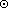star ejects its envelope. [H76] Redshift The shift of spectral lines toward longer wavelengths in the spectrum of a receding source of radiation. [Silk90] (b) If a star or galaxy is moving away from us, the radiation from the star or galaxy appears shifted towards longer wavelengths, or towards the red end of the spectrum. See Doppler shift. [G97] (c) The shift to the red of a star's spectrum caused by the star's movement away from us. This movement stretches the star's light waves and increases their wavelength. Since red has a longer wavelength than blue, this shift is called a redshift. The larger a star's redshift, the faster the star is moving away from us. Most galaxies also show redshifts, not because of the galaxy's movement away from us (although the galaxy is moving away from us) but because of the expansion of the universe. As a galaxy emits a light wave toward us, the light wave travels through the fabric of space; en route to Earth, it is stretched by the expansion of space and exhibits a redshift. The farther the galaxy, the larger the redshift. To distinguish this type of redshift from one caused by movement, astronomers call it the "cosmological redshift". [C95] (d) The shift of spectral lines toward longer wavelengths, either because of a Doppler effect or because of the Einstein effect (gravitational redshift). The redshift z =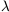/whereis the laboratory wavelength of the spectral line andis the difference between the laboratory and the observed wavelengths. The redshift of distant galaxies was first noted by Slipher in 1926. [H76] Redshift-Distance Relation The correlation between redshift in the spectra of galaxies and their distances. See Hubble law. [F88] Redshift-Magnitude Test A cosmological test involving the plotting of redshifts and apparent magnitudes of distant galaxies. Deviations from the relation expected in Euclidean space can help determine whether the universe is open or closed. The redshift-magnitude test is very sensitive to evolutionary effects (whether galaxies were brighter or dimmer in the past). [Silk90] Redshift Survey The methodical tabulation of the redshifts of a large number of galaxies in a particular region of the sky. Redshifts directly measure the recessional speeds of galaxies. If Hubble's law is assumed, this speed can be translated to distance. Under such an assumption, a redshift survey provides the third dimension, depth, for the galaxies in a survey. The other two dimensions for each galaxy are provided by its position on the sky. The redshift of a galaxy is obtained by measuring its spectrum of light; in this way it is possible to see how much its colors are shifted. (See spectrum.) [LB90] Red Spot An elliptical spot about 40,000 × 15,000 km on the southern hemisphere of Jupiter. Its color and intensity vary with time. It has been observed for at least a century, and an examination of earlier records shows that Cassini had sketched it in the seventeenth century. [H76] Red Supergiant A supergiant with spectral type M. Red supergiants are the largest stars in the universe: if put in place of the Sun, some would touch Saturn. The two brightest red supergiants in Earth's sky are Betelgeuse and Antares. [C95] Reduced Proper Motion The observed proper motion of a star (in seconds of arc per year) reduced to absolute proper motion (in kilometers per second). [H76] Reflecting Telescope Telescope that uses mirrors to magnify and focus an image onto an eyepiece. (reflector) [A84] Reflection Nebula A cloud of interstellar gas and dust whose spectrum contains absorption lines characteristic of the spectrum of nearby illuminating stars. The emission component of its spectrum is due to gas; the reflection component, to dust (see also diffuse nebula). [H76] Refracting Telescope Telescope that uses lenses to magnify and focus an image onto an eyepiece. (refractor) [A84] Refraction, Astronomical The change in direction of travel (bending) of a light ray as it passes obliquely through the atmosphere. As a result of refraction, the observed altitude of a celestial object is greater than its geometric altitude. The amount of refraction depends on the altitude of the object and on atmospheric conditions. [S92] Refraction, Law of Snell's law. [H76] Refraction, Refractive Index (a) Deflection (or "bending") of light - or any ray as it passes from one medium into another of greater or lesser density, representing a change in overall speed of the ray. Refracting telescopes rely on the refraction of light through lenses. The refractive index of a medium (e.g., glass) is a measure of the medium's "bending" power. [A84] (b) A number which characterizes the properties of a material when light is transmitted through it. A vacuum has a refractive index of 1.0; air is fairly close to this also. As the refractive index rises, light waves travel more slowly in the material, more light is reflected and the bending or refraction of light is greater. Some materials have a different refractive index depending on the plane of vibration of the electromagnetic wave, they are called birefringent. [McL97] Refractor A telescope in which the light is focused by a lens at the viewing side of the telescope. By contrast, a reflecting telescope is one in which light is focused by a mirror. [LB90] Regge Trajectory Derived from S-matrix theory, the Regge Trajectories were theoretical plots that attempted to account for the position of elementary particle resonances. One of the triumphs of early string theory was to describe the general shape of these Regge Trajectories. [P88] Regge-Wheeler Equations Schrödinger-type equations for small, odd- parity perturbations on the Schwarzschild metric. [H76] Regolith The layer of fragmentary debris produced by meteoritic impact on the surface of the Moon or a planet. [H76] Regression of the Nodes The slow (19°.35 per year, 360° in 18.6 years), westward motion of the nodes of the Moon's orbit due to perturbations of the Earth and Sun. [H76] Regulus (Leo) A visual triple B8 V star about 26 pc distant. [H76] Relative Number See Wolf number. [H76] Relativistic Approaching the velocity of light. Particles moving at these speeds demonstrate effects predicted by the special theory of relativity - increased mass, slowing of time, etc. - that must be taken into account by combining relativity with quantum theory if accurate predictions are to be made. [F88] Relativistic Beaming Theory devised by Francis Smith regarding the generation of polarized radiation in neutron stars (pulsars). [A84] Relativistic Bremsstrahlung Hypothetical gravitational radiation emitted when two stars fly past each other with high velocity and deflect each other slightly. [H76] Relativistic Cosmology Cosmological applications of Einstein's theory of general relativity. The Big Bang cosmological models were first derived according to the equations of relativistic cosmology, which are needed for many applications of these models. [Silk90] Relativistic Particles Particles whose velocities approach the speed of light. [Silk90] Relativistic Quantum Field Theory Quantum-mechanical theory of fields, such as the electromagnetic field, that incorporates special relativity. [G99] relativistic plasma a plasma consisting of particles which have relativistic energies, i.e. for typical particles, their kinetic energies exceed their rest-mass-energies mc2. Relativistic plasmas are found in such astronomical objects as supernova remnants, radio galaxies, the interstellar medium and the nuclei of galaxies. In many of these cases, the relativistic plasma provides most of the pressure of the medium. The spectrum of the particle energies is generally of power-law rather than Maxwellian form so that many of the particles are ultrarelativistic, i.e. E >> mc2. [D89] Relativistic Zone For a pulsar, the region in which M[grams] / R[cm] is not negligible compared with unity. [H76] Relativity (a) The theory of how motion and gravity affect the properties of time and space. The special theory of relativity establishes, among other things, the nonabsolute nature of time. The amount of time elapsed between two events will not be the same for two observers or clocks in relative motion to each other. The general theory of relativity describes how gravity affects the geometry of space and the rate at which time passes. (See general relativity; special relativity.) [LB90] (b) The special theory concerns time and distance measurements by two observers in uniform relative motion, and clarifies the notion of simultaneity relative to such observers. The general theory of relativity is concerned with the generalization of Newton's law of gravitation when masses moving under their mutual influence acquire velocities comparable to that of light; its basic postulate, derived from the equality of the inertial and the gravitational mass, is that all accelerations are metrical in origin. [H76] Relaxation The process of gravitational interaction (in the case of a cluster of stars or galaxies) whereby a random distribution of motions is eventually established. The system is said to relax to a state of thermal equilibrium. [Silk90] Relaxation Time Period required for the reestablishment of thermal equilibrium; in particular (in the astronomical context) the period required for the reestablishment of a random distribution of motion in a cluster of stars. [H76] renormalisation strictly, the rescaling of some parameter in a field theory. In practice, nearly all renormalizations involve an infinite rescaling, so the term has come to be identified with a mathematical procedure for circumventing otherwise nonsensical infinite terms in quantum field theory by absorbing them into observable constants in the theory, such as mass, charge, etc. [D89] Renormalization (a) The mathematical process which ensures that the basic quantities in quantum field theory (e.g. in QED: the photon, electron and electric charge) are well-defined and not infinite. [CD99] (b) A mathematical procedure in quantum mechanics that allows one to make a correspondence between the formal quantities of the theory and the actual quantities observed in the lab. Some of the formal quantities, such as the mass of an electron, have infinite values before renormalization. [LB90] (c) When a theory such as quantum electrodynamics is approximated by a perturbation expansion, the first approximation is found to give answers that agree well with experiment. The second approximation, however, is found to produce mathematical expressions that are infinite, and hence meaningless. Renormalization is a technique for reformulating the theory so that the infinities are avoided, developed independently by Feynman, Tomonaga, and Schwinger in the 1940s. [G97] renormalisation group the way in which coupling constants enter into field theory often involves certain simple scaling relations that are described by a group (in the mathematical sense), In statistical mechanics, the renormalization group method systematically implements some form of coarse-graining operation to expose the character of the large-scale phenomena, in physical systems where many scales are important. [D89] Reproducing Universes The process in some inflationary universe models whereby the universe is constantly spawning new universes, causally disconnected from each other and from the parent universe. (See chaotic inflation.) [LB90] Reseau A grid that is photographed by a separate exposure on the same plate with star (or galaxy) images. [H76] Reset Noise The unwanted and uncertain electrical signal transmitted to the output pin of a CCD during the process of recharging, via the reset transistor, the output storage capacitor to its preset value in readiness for the next pixel charge. [McL97] Residual Intensity Ratio of correlated flux in the line to correlated flux in the continuum. [H76] Resolution The ability of an optical system, including detector, to separate two adjacent objects - this is called "spatial resolution" - or two adjacent wavelengths in a spectrometer - this is called "spectral resolution". [McL97] Resolving Power (a) The ratio of the mean wavelength of two lines to the minimum resolvable angle. The resolving power of the human eye is about 1 minute of arc (its integration time is about 1/15 second). [H76] (b) The ratio of the wavelength of radiation to the smallest interval of wavelength that the instrument can measure. [McL97] Resonance (a) One of the natural states of oscillation of a physical system. [G99] (b) The selective response of any oscillating system to an external stimulus of the same frequency as the natural frequency of the system. Under such conditions the nodes of the two wave trains coincide, and the waves of the initial system increase in amplitude. The natural mode of oscillation of a star varies inversely as the square root of its mean density; if the Sun were to start resonating at its natural frequency, it would have a period of about an hour. [H76] (c) Resonances are sometimes found when elementary particles collide and interact together. They represent tiny regions of space in which energy is temporarily bound. [P88] Resonance Capture Capture by an atomic nucleus of a particle whose energy is equal to one of the energy levels of the nucleus. Under such circumstances the particle's chances of being captured are greatly increased. [H76] Resonance Line The longest-wavelength line arising from the ground state. [H76] Resonance Particles (a) Hadronic particles which exist for only a very brief time (10-23 seconds) before decaying into hadrons. (also called resonances) [CD99] (b) Strongly interacting particles which are born and decay within the short time span of the strong interaction (10-23 seconds). The existence of a resonance cannot be observed directly; it can only be inferred from studying the longer-lived products of its decay. An asterisk is commonly used to designate a resonance, e.g.,*. [H76] Resonant Reaction A nuclear reaction that has an energetically favorable probability of occurring (see resonance capture). [H76] Rest-Mass Energy The energy which a particle has even when it is at rest. According to the famous relation E = mc2 of special relativity, this rest energy is equal to the rest mass of the particle-the mass it has when a rest-times the square of the speed of light. If the mass is in grams and the speed of light in centimeters per second (c = 2.998 × 1010 centimeters per second), then the energy is given in ergs. [G97] Restoration A process used by radio astronomers to eliminate the smoothing effect observed in radio maps that is caused by the finite width of the telescope beam. [H76] Retarder A device for introducing a phase delay, such as half-wave or quarter-wave, between two orthogonally polarized components of an electromagnetic wave. [McL97] Reticle A system of cross-hairs in the eyepiece of a telescope. [H76] Retrograde (a) In a backwards direction; in astronomy this means in a direction corresponding to east-to-west. [A84] (b) Apparent motion of a planet in a direction opposite to its normal progress across the sky produced by the orbital motion of the earth. [F88] Reversing Layer Lower chromosphere of the Sun, a comparatively cool region in which radiation at certain wavelengths is absorbed from the continuous spectrum emitted from the Sun's photosphere. [A84] Reynolds Number (a) A dimensionless number (R = Lv/, where L is a typical dimension of the system, v is a measure of the velocities that prevail, andis the kinematic viscosity) that governs the conditions for the occurrence of turbulence in fluids. [H76 (b) The Reynolds number is one of the more important of the non-dimensional parameters of fluid motion. In 1883 Osborne Reynolds (1842-1912) showed the flow of a fluid in a tube could be described by a dimensionless constant. This constant, which is now called Reynolds' number, is given by vl /, where l has the dimensions of length, v is the fluid velocity andis the kinematic viscosity. Subsequent work has extended Reynolds' ideas to all cases of fluids in motion. In general low values of Re show that the viscous forces are predominant in controlling the flow whereas higher values indicate that the inertial forces are more important. When the fluid is moving through a curved pipe its flow continues to be governed by the Reynolds number, but in this case its numerical value is changed by taking into account the ratio of cross section (R) and of curvature (r) of the tube. This derived constant is sometimes called the Dean number and is equal to 1/2Re(R/r)1/2. The movement of a fluid between two rotating cylinders of radii R1 and R2 may be described by a dimensionless constant based on Reynolds' number and known as the Taylor number (Ta), where Ta = 2Re2(R1 - R2) / (R1 + R2). [JM92] RF Power Radio-Frequencypower. Electromagnetic fields alternating at the frequencies of radio waves (up to 1010 Hz), which can be used to accelerate charged particles in accelerators. [CD99] Rhea Sixth satellite of Saturn, discovered by Cassini in 1672. Diameter about 1500 km; rotation period 4d12h25m. Albedo 0.57. [H76] RheniumRhodiumRich Clusters Clusters with a particularly large number of galaxies. [LB90] Richardson-Lucy Method An image reconstruction algorithm. [McL97] Riemannian Geometry A large class of non-Euclidean geometries. The mathematics of general relativity uses Riemannian geometry. (See general relativity.) [LB90] Riemannian Geometry Mathematical framework for describing curved shapes of any dimension. Plays a central role in Einstein's description of spacetime in general relativity. [G99] Rigel (a) A blue supergiant some 900 light-years away in the constellation Orion. Rigel is the brightest star in Orion and the seventh brightest star in the night sky. [C95] (b) A B8 Ia supergiant (Ori) at least 400 pc distant, the brightest star in Orion. It is a multiple star. [H76] Right Ascension Angular distance on the celestial sphere measured eastward along the celestial equator from the equinox to the hour circle passing through the celestial object. Right ascension is usually given in combination with declination. [S92] Ring Galaxy A galaxy with a ring-like appearance. The ring contains luminous blue stars, but relatively little luminous matter is present in the central regions. It is believed that such a system was an ordinary galaxy that recently suffered a head-on collision with another galaxy. [Silk90] Ring Nebula A famous planetary nebula (M57, NGC 6720) in the constellation Lyra. [C95] Rise Time The time required for the vehicle to achieve its optimum height (in rocket or balloon astronomy). [H76] Ritchey-Chrétien (a) A class of reflecting telescope with a hyperbolic primary and secondary. [McL97] (b) A system of two mirrors, aspherized to give an image at the secondary (Cassegrain) focus free from spherical aberration and coma. [H76] Ritz Combination Principle A principle discovered empirically before the advent of quantum mechanics which states that every spectral line of a given atom corresponds to the difference of some pair of energy levels.[H76] rms root mean square. [LLM96] Robertson-Walker Metric (a) An equation which describes the spacetime continuum in a Universe which adheres to the cosmological principle. [c97] (b) A metric which is appropriate for a spacetime which is homogeneous and isotropic. It was derived by Robertson and Walker with a minimum of assumptions; but the metric had been used earlier by Friedmann to derive the cosmological models (without a cosmological constant) that are currently in use. [H76] (c) A mathematical description of the geometrical properties of a homogeneous and isotropic universe. Friedmann cosmologies all use the Robertson-Walker metric. [LB90] Rocard Scattering Linearly anisotropic scattering. [H76] Roche Limit The minimum distance at which a satellite under the influence of its own gravitation and that of a central mass about which it is describing a circular Keplerian orbit can be in equilibrium. For a satellite of negligible mass, zero tensile strength, and the same mean density as its primary, in a circular orbit around its primary, this critical distance is 2.44 times the radius of the primary. (For the Moon, whose density is lower than that of Earth, the Roche limit would be 2.9 Earth radii.) [H76] Roche Lobe The first equipotential surface for two massive bodies describing circular orbits around one another which forms a figure eight enclosing the two objects. The Roche lobes are the two lenticular volumes enclosing the two bodies. [H76] Rods The more sensitive cells of the retina of the eye. They are of most importance in vision in poor light, but cannot provide color information. Their action is not known in detail. It appears to be based on the photochemical breakdown of a reddish dye called rhodopsin (formerly visual purple). [DC99] Root Mean Square (rms) 1. The square root of the average of the squares of a group of numbers or values. For example, the RMS value of {2, 4, 3, 2.5} is [22 + 42 + 32 + 2.52)/4]1/2 = 5.937 2. For continuous quantities, such as alternating electric current, the root-mean-square value is used to measure the average effect. The root-mean-square value of an alternating current (also called the effective value) is the value of the direct current that would produce the same energy transfer per second in a given resistor. For a sine wave, this is Im/21/2, where Im is the peak value. [DC99] Root Mean Square (rms) The square root of the mean square value of a set of numbers. [H76] ROSAT ROentgen SATellite, was an X-ray observatory developed through a cooperative program between the Germany, the United States, and the United Kingdom. The satellite was designed and operated by Germany, and was launched by the United States on June 1, 1990. It was turned off on February 12, 1999. Rosette Nebula An HII region (M16, NGC 2237-2244) in Monoceros, more than 1 kpc distant. It has a fairly high degree of symmetry about a centrally located cluster of about six hot, young O-type stars. [H76] Rossby Number This gives the ratio of the inertial to the Coriolis forces in a moving fluid. It is named after the Swedish meteorologist C. A. Rossby (1899-1959). [JM92] Rossby Waves Cyclonic convection waves in a rotating fluid. Such waves occur in the atmosphere, in the oceans, and in the fluid core of the Earth. [H76] Rosseland Mean Absorption Coefficient A coefficient of opacity which is a weighted inverse mean of the opacity over all frequencies. It is applied when the optical depth is very large. [H76] Rossiter Effect A rotational distortion of the velocity curves of eclipsing binaries. [H76] Rotation Of a single body in space: spinning on an axis. Of a planetary system, rotation is generally planar in relation to the parent star. Galactic rotation, however, is usually differential. [A84] Rotation Curve A quantitative description of how fast each part of a galaxy is rotating about the center. A rotation velocity is the velocity of a rotating galaxy at a certain distance from the center of the galaxy. A "flat" rotation curve is one in which the rotational velocity of the galaxy remains constant with distance away from the center of the galaxy. This indicates that the mass of the galaxy increases linearly with distance from its center. (See Keplerian rotation curve.) [LB90] Rotational Invariance The property of being unchanged by a rotation. A sphere is rotationally invariant, but a rectangle is not. As far as we know the fundamental laws of physics are rotationally invariant. [G97] Rotational Transition A slight change in the energy level of a molecule due to the rotation of its constituent atoms about their center of mass. [H76] Royal Greenwich Observatory RGO: Primary national 0bservatory in Great Britain, first sited at Greenwich in 1675, but in 1958 moved to Herstmonceux, Sussex. From the first, Directorship of the Observatory has entailed appointment as Astronomer Royal. In the 1980s the Observatory will lose its primary national status with the completion of the Northern Hemisphere Observatory in Las Palmas, the Canary Islands. [A84] Royal Society English organization founded in the seventeenth century and dedicated to the advancement of science. [F88] r-Process The creation of elements heavier than zinc through the rapid bombardment of other elements by neutrons. The r process occurs in supernovae. Examples of reprocess elements are gold, iodine, and europium. [C95] RR Lyr (a) Periodic variables with periods less than one day, and of spectral types A to early F. [JJ95] (b) Type of short-period variable stars. Spectrally classified as A to F giants. They were once called cluster-cepheids. [A84] (c) An old metal-poor white or yellow-white giant star that pulsates like a Cepheid and therefore varies in brightness. Most RR Lyrae stars have periods of under one day, which is shorter than periods for Cepheids. RR Lyrae stars are also fainter than Cepheids, with absolute magnitudes around +0.7, corresponding to a luminosity about 45 times the Sun's. RR Lyrae stars are excellent distance indicators because they all have nearly the same intrinsic brightness. They take their name from the star RR Lyrae, in the constellation Lyra. [C95] RS CVn Stars Close binaries, which show H and K emissions. [JJ95] RTI Rise Time Invariance. RubidiumA soft silvery highly reactive element. Naturally occurring rubidium comprises two isotopes, one of which, 87Rb, is radioactive (half-life 5 × 1010 years). The element is used in vacuum tubes, photocells, and in making special glass. Symbol: Rb; m.p. 39.05°C; b.p. 688°C; r.d. 1.532 (20°C); p.n. 37; r.a.m. 85.4678. [DC99] Runaway Stars (a) Early type stars (O and early B) outside the Galactic plane, which reached large distances (from the Galactic plane) because of their high velocities. [JJ95] (b) Stars of spectral type O or early B with unusually high space velocities. The three best known are AE Aur, 53 Ari, and µ Col, all of which diverge from a comparatively small area in Orion. Runaway stars are thought to be produced when there is a supernova explosion in a close binary system. [H76] Runge-Kutta Method A step-by-step method of numerical integration. [H76] Russell-Saunders Coupling See LS coupling. [H76] Russell-Vogt Theorem See Vogt-Russell theorem. [H76] RutheniumA transition metal that occurs naturally with platinum. It forms alloys with platinum that are used in electrical contacts. Ruthenium is also used in jewelry alloyed with palladium. Symbol: Ru; m.p. 2310°C; b.p. 3900°C; r.d. 12.37; p.n. 44; r.a.m. 101.07. [DC99] Rutherfordium A radioactive metal not found naturally on earth. It is the first transactinide metal. Atoms of rutherfordium are produced by bombarding 249Cf with 12C or by bombarding 248Cm with 18O. Symbol: Rf; m.p. 2100°C (est.); b.p. 5200°C (est.); r.d. 23 (est.); most stable isotope 261Rf (half-life 65s). [DC99] RV Tau Variables Periodic variables with periods 60-100 days, and of spectral types G and K. [JJ95] Rydberg A unit of energy (R =3c / me4) equal to 13.5978 eV (the ionization potential of hydrogen). Rydberg atom extremely highly excited atoms are called Rydberg atoms (Rydberg was an early systematizer of atomic spectra). These atoms are huge (the size of viruses for the largest) and fragile but interact very strongly with radiation. Quantum opticians use them to test basic ideas about atom-photon interactions.[D89] Rydberg Correction See Quantum Defect. [H76] Rydberg Formula An equation that gives the wave number of a line in a spectrum: 1 /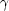= R(1 / n2 - 1 / m2) where n and m are positive whole numbers and R is the Rydberg constant (equal to 1.096 77 × 107 m-1 for hydrogen). [DC99] Rydberg Formula A formula by which the various lines in a given spectral series are obtained:-1 = R[(m + k1)-2 - (n + k2)-2], where m is an integer, n is any integer greater than m, k1 and k2 are empirical corrections which are different for different series, and R is the Rydberg constant, which has a value of 109,678 ifis measured in centimeters. [H76]